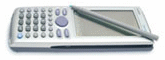# G-solve

4 replies to this topic

### #1fiberoptik

fiberoptik

•• Members
•• 70 posts

• Calculators:
ClassPad 300, FX-850P, FX-7700G, Voyage 200

Posted 04 November 2004 - 11:44 AM

Hi people,
entering the expression (2x^2+6x+1)/(x^2+1), and then asking for the inflection point the CP returns : ERROR: Can't Solve.
Now if i make diff(2x^2+6x+1/x^2+1,x,2) it gives me the 2nd derivative, then i drag the result to graph window. next i graph the first function, the intersection of the two graphics are the inflection points. With pencil and paper, to find the inflections points we need to find the roots of the 2nd derivative( the roots are the x value of the inflection point), thats what i did graphically, my questiion is : if the CP can calculate the 2nd derivate why can it calculate the inflections points of the first expression directly ? I hope i made my self clear enough.

Thanks

### #2Lovecasio

Lovecasio

Casio Freak

•• Members
•• 242 posts
• Location:Hochiminh city Vietnam
• Interests:Organic chemistry.<br />Pharmacy

• Calculators:
fx 570 MS, Casio AFX 2.0+, ClassPad 300

Posted 04 November 2004 - 12:34 PM

Hi.
Inflection is the point where the graph change from comvex to concave ( or inverse ). Let y=f(x) which has graph ©. I is the inflection point when f''(x) change its sign when it "passes" xi. I'm sorry but I'm in disagreement with you because you said that " the intersection of the two graphics are the inflection points " and " the roots of the 2nd derivative( the roots are the x value of the inflection point) ". I haven't tried yet, but you can adjust ViewWindows and then solve it again.
Tell me if I said anything wrong.

### #3fiberoptik

fiberoptik

•• Members
•• 70 posts

• Calculators:
ClassPad 300, FX-850P, FX-7700G, Voyage 200

Posted 04 November 2004 - 03:39 PM

Hi, maybe i didn't make myself clear enough.
To find the inflection points manually we must find the f''(x), then we find the roots of f"(x), this roots are the x coordinate (x,y) of the inflection point, then with this value (x) we substitute in the original expression and get y coordinate of the inflection point. As for " the intersection of the two graphics are the inflection points " i was wrong, a said that because when i draw the two graphics the intersections were very near of the inflection point. You can try with for example (x^3)+1, draw this function and then draw the f''(x), you 'll see that the intersection is near the real inflection point. Visually, we could eventually think that the intersection would be de inf point, but it isn't. I'll try to change ViewWindow.

### #4Lovecasio

Lovecasio

Casio Freak

•• Members
•• 242 posts
• Location:Hochiminh city Vietnam
• Interests:Organic chemistry.<br />Pharmacy

• Calculators:
fx 570 MS, Casio AFX 2.0+, ClassPad 300

Posted 05 November 2004 - 05:49 AM

Hi.
Not always are roots of f''(x) the x-coor of inflection points.
Example: y=x^4 ( C )
We have y'=4x^3
y''=12x^2
Then, y''=0 <=> x=0.
However, the point (0;0) is not the inflection point of ( C ) since y'' doesn't change it 's sign as it passes x=0.

### #5fiberoptik

fiberoptik

•• Members
•• 70 posts

• Calculators:
ClassPad 300, FX-850P, FX-7700G, Voyage 200

Posted 05 November 2004 - 10:43 AM

Is not an inflection point because x^4 DON'T have inflection points! X^4 is a parabola, so the concavity don't change (always up or always down). The points of inflection tell us where the concavity changes. You can be shure that the points of inflection are calculated the way i told you before. Check this link, it has an exmple with x^4 . Anyway thank you for your answers.

http://www.clas.ucsb...tion Points.htm

#### 0 user(s) are reading this topic

0 members, 0 guests, 0 anonymous users